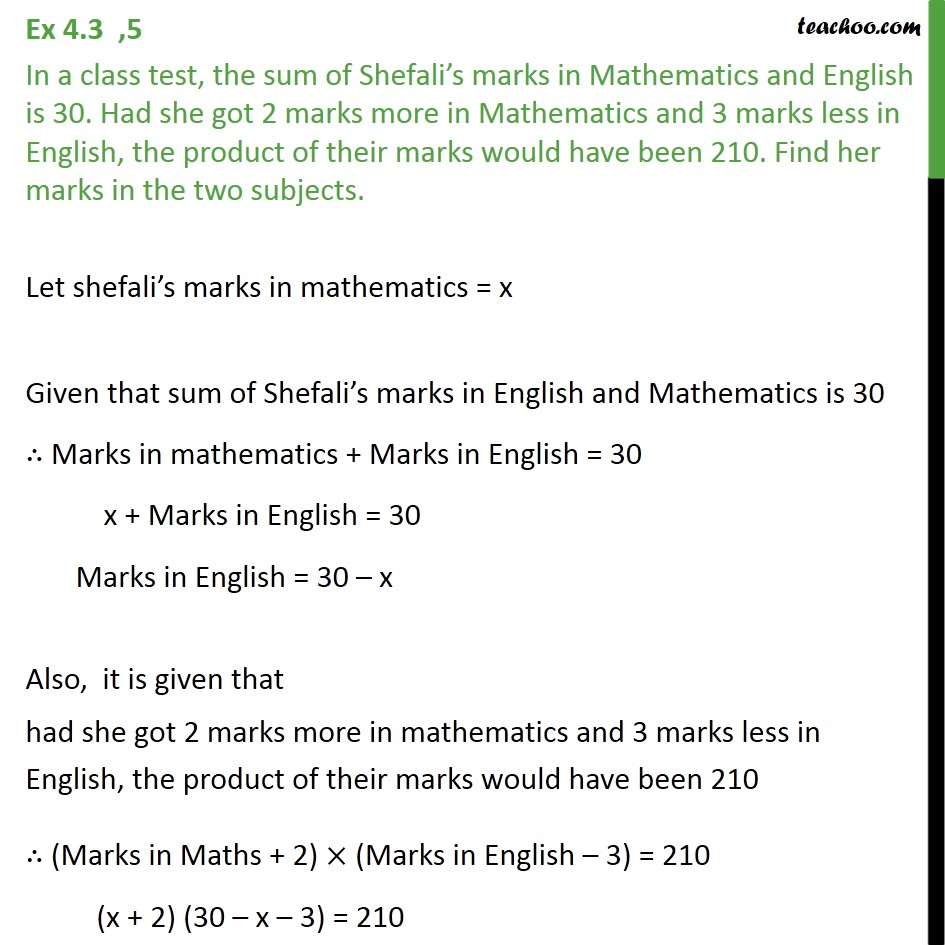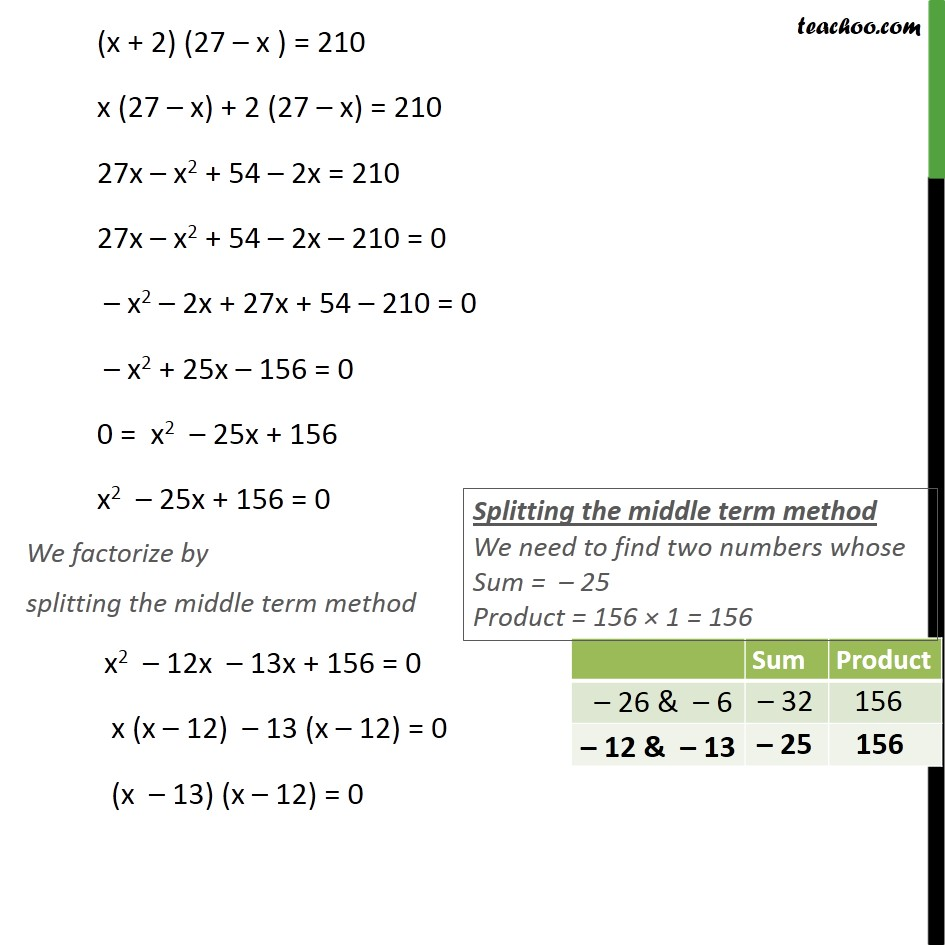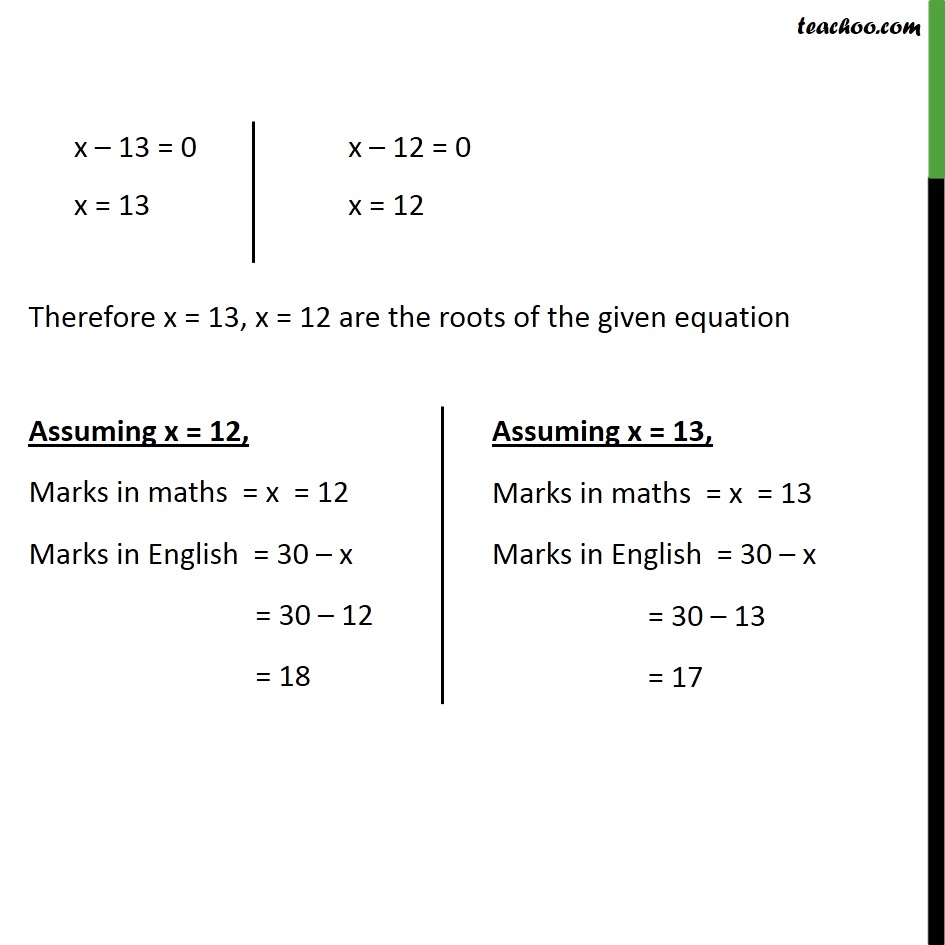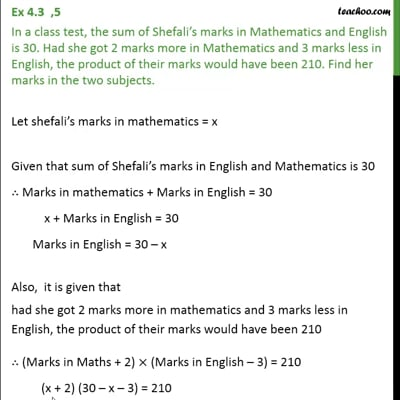Ex 4.3

Chapter 4 Class 10 Quadratic Equations
Serial order wiseThis video is only available for Teachoo black users

Solve all your doubts with Teachoo Black (new monthly pack available now!)

### Transcript

Ex 4.3 ,5 In a class test, the sum of Shefali s marks in Mathematics and English is 30. Had she got 2 marks more in Mathematics and 3 marks less in English, the product of their marks would have been 210. Find her marks in the two subjects. Let shefali s marks in mathematics = x Given that sum of Shefali s marks in English and Mathematics is 30 Marks in mathematics + Marks in English = 30 x + Marks in English = 30 Marks in English = 30 x Also, it is given that had she got 2 marks more in mathematics and 3 marks less in English, the product of their marks would have been 210 (Marks in Maths + 2) (Marks in English 3) = 210 (x + 2) (30 x 3) = 210 (x + 2) (27 x ) = 210 x (27 x) + 2 (27 x) = 210 27x x2 + 54 2x = 210 27x x2 + 54 2x 210 = 0 x2 2x + 27x + 54 210 = 0 x2 + 25x 156 = 0 0 = x2 25x + 156 x2 25x + 156 = 0 We factorize by splitting the middle term method x2 12x 13x + 156 = 0 x (x 12) 13 (x 12) = 0 (x 13) (x 12) = 0 Therefore x = 13, x = 12 are the roots of the given equation Assuming x = 12, Marks in maths = x = 12 Marks in English = 30 x = 30 12 = 18